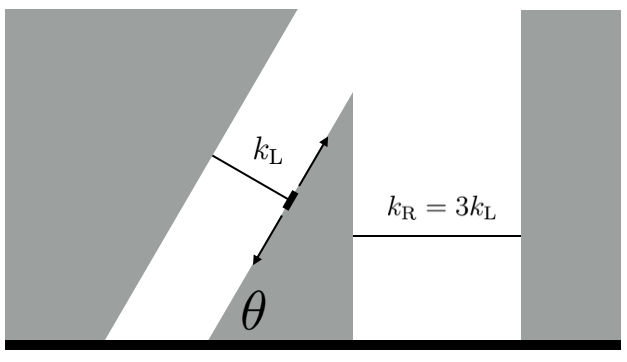# Simple Harmonic Motion wedgeA smooth wedge of mass $m$ and angle of inclination $\theta = \SI{60}{\degree}$ is attached to two springs of spring constant $k_\textrm{L}$ on the left, and $k_\textrm{R} = 3k_\textrm{L}$ on the right. The wedge rests on a smooth frictionless plane. Find the period of oscillation of the wedge in seconds.

Give your answer to 3 decimal places.

Details and Assumptions:

• The springs are perpendicular to the respective sides they are facing.
• The spring on the left is constrained to compress and extend along its length. It is attached to the wedge by a frictionless roller that can move along the hypotenuse.
• $m=\SI{3}{\kilo\gram}$
• $k_\textrm{L}= \frac18 \si[per-mode=symbol]{\newton\per\meter}$.
###### Try my set.
×

Problem Loading...

Note Loading...

Set Loading...